### Home > CALC > Chapter 1 > Lesson 1.2.4 > Problem1-70

1-70.
1. Selected values of a continuous even function are shown below. 1-70 HW eTool (Desmos). Homework Help ✎

 x f(x) 0 1 2 3 0 2 4 6

1. Find f(−l), f(−2) and f(−3).

2. Sketch a possible graph of f(x) on the domain −3 ≤ x ≤ 3.

3. Sketch another possible graph of f(x) on the domain −3 ≤ x ≤ 3.

4. Could the graph of f(x) be a parabolic function? If so, find a possible equation of f(x). If not, explain.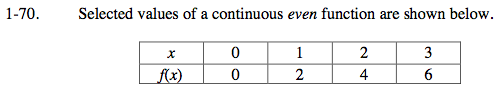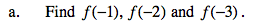Defintion of an even function: f(−a) = f(a).

Since f(1) = 2 then, by definition, f(−1) = 2 as well.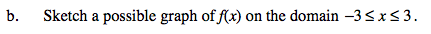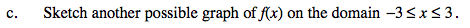There are many ways to connect the points, just make sure you maintain symmetry over the y-axis.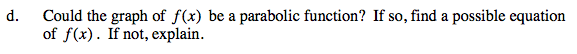Can you draw a parabola through all of these points? Give it a try.

Use the eTool below to view the graphs of some possible functions.
Click the link at right for the full version of the eTool: Calc 1-70 HW eTool